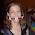## Thursday, March 26, 2009

### Six Sides Faceup

With a standard six-sided die, how many throws are required on average before each of the six numbers has landed faceup?
1. 10
2. 15
3. 20
4. 25

1.1 throw for the first number
6/5 of a roll for the second unique number
3/2 of a roll for the third unique number
2 rolls for the fourth
3 rolls for the fifth
6 rolls for the last

Add those up for an expected value of 14.7 rolls (round to 15, I suppose).

I used a sort of back-door method to solve this, but it seems reasonable. The expected value of number of rolls to get your next unique number is just an infinite sum of the possible values times the probability that that value occurs.

For example, the first roll gives some number. Then, on your second roll, you have a 5/6 chance of rolling a new number on 1 roll. You have a 1/6*5/6 chance of rolling a new number in 2 rolls (1/6 to roll the same number times 5/6 to roll any of the remaining 5 on the next roll). By the same logic, you have a (1/6)^2*5/6 chance to roll. So the average number of rolls to get your second unique number is the sum of the roll number times the probability of that number occuring, which is the sum from n = 1 to infinity of n*(1/6)^(n-1)*5/6.

I hope that makes sense...

2.One more thing...the sum of these infinite series comes out to be those numbers at the top. You can find it pretty easily (relatively, I guess) by playing around with the formula for an infinite geometric series, taking the derivative with respect to n, etc. Or use Mathematica or something.

3.Well, i figured out that the chance of getting it in 6 rolls would be 1.5%... very low.

4.That sounds absolutely right, Abe.

I actually misunderstood this question and ended up calculating a different number. I wouldn't have thought it would be a different value, but now that I think about it makes sense.

I was looking for the first number of rolls that produced a greater than fifty percent chance of yielding 6 unique values. I took an inductive approach, then sort of cheated by letting EXCEL do the work for me:

I figured that if you're thinking of the case of n rolls, you could compute the odds of having at least m unique values if you knew two things about the n-1 rolls case: The probability that you had at least m unique values in that case (call this quantity G); and the probability that you had at least m-1 unique values in that case (call this quantity P). In order to have m unique values in the n rolls scenario, you'd have to have fulfilled one of these two cases in the n-1 rolls case scenario. You produce a value of at least m with n dice in iterations in which 1)You already had at least m (probability: G), or 2)You had exactly m-1 (probability: P-G), and then you produced a new unique value (probability: (7-m)/6). So the total probability of having m unique values with n dice is (P-G)*(7-m)/6 + G, in terms of these quantities.

I cheated by calculating this inductively from two base cases, using EXCEL: The chances of having at least 1 unique value with any amount of rolls (1), and the chances of having "at least" any number of unique values above 1 with one roll (0). I hard-coded those values in column B and row 2 (allowing for labels), then pasted the following into cell C3:
=(B2-C2)*(8-COLUMN(C\$1))/6+C2
and pasted down and right through the table.
This formula gains credence from the fact that, were it extended to a seven-dice column, it's value would always be zero.

The resulting spreadsheet (or at least the values) can be found at the following link:

Since 0.5 is passed for 6 values once you hit 13 dice, you'd get 6 different values more than 50% of the time with thirteen dice.

So, if you're asked to lay money on a certain number of dice rolls, you're likely safe at 13. If you're asked instead to lay money on the number of rolls it will take to hit that sixth value, though, you should up it to 15.

Similarly, if you're betting you'd get two different values in two coin flips, you're at a dead heat. If you're betting how many flips it will take to get the second value, though, an extension of Abe's formula will lead you (correctly) to bet that it will take 3 flips on average.

5.6.Yeah, I think expected value calculations are the way to go with this sort of problem, n(avg.) = Sum (n*P(n)).

Leave your answer or, if you want to post a question of your own, send me an e-mail. Look in the about section to find my e-mail address. If it's new, I'll post it soon.

Please don't leave spam or 'Awesome blog, come visit mine' messages. I'll delete them soon after.

Enter your Email and join hundreds of others who get their Question of the Day sent right to their mailbox

The Lamplight Manor Puzz 3-D